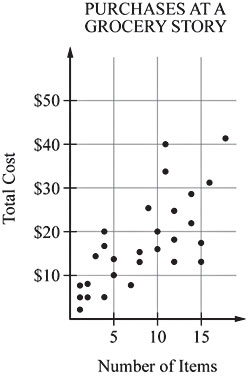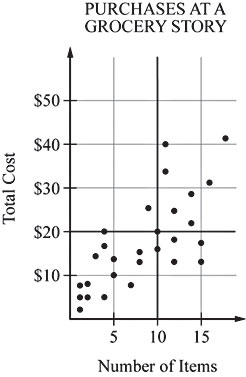# SAT官方网站每日一题系列(88)评论打印收藏本文The scatterplot above shows the number of items purchased at a grocery store by 28 customers and the total cost of each purchase. How many of these 28 customers bought more than 10 items and spent less than \$20?

A. Four

B. Five

C. Six

D. Seven

E. Eight

Drawing the vertical line "Number of Items = 10" and the horizontal line "Total Cost = "\$20" divides the scatterplot into four regions, as shown in the figure below.The lower right region lies below the line "Total Cost = \$20" and to the right of the line "Number of Items = 10." Thus the points in this region are exactly the points that represent a purchase of more than 10 items at a total cost of less than \$20. (Note the that points on the line "Number of Items = 10" represent a purchase of exactly 10 items, not more than 10 items and, thus, do not meet the given conditions.) There are four points in the lower right region. Therefore, four of the customers bought more than 10 items and spent less than \$20.

 重点单词 查看全部解释 multiple ['mʌltipl] 想一想再看 adj. 许多，多种多样的n. 倍数，并联 联想记忆 horizontal [.hɔri'zɔntl] 想一想再看 adj. 水平的，横的n. 水平线，水平面 联想记忆 vertical ['və:tikəl] 想一想再看 adj. 垂直的，顶点的，纵向的n. 垂直物 联想记忆 purchase ['pə:tʃəs] 想一想再看 vt. 买，购买n. 购买，购买的物品 figure ['figə] 想一想再看 n. 图形，数字，形状; 人物，外形，体型v 联想记忆
﻿# 可可英语官方微信(微信号:ikekenet)

每天向大家推送短小精悍的英语学习资料.添加方式1.扫描上方可可官方微信二维码。
添加方式2.搜索微信号ikekenet添加即可。